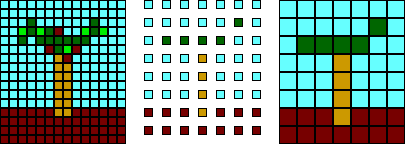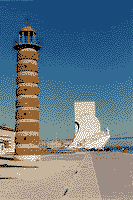## Intraframe Compression Techniques

Intraframe compression is compression applied to still images, such as photographs and diagrams, and exploits the redundancy within the image, known as spatial redundancy. Intraframe compression techniques can be applied to individual frames of a video sequence.

### Sub-sampling

Sub-sampling is the most basic of all image compression techniques and it reduces the amount of data by throwing some of it away. Sub-sampling reduces the number of bits required to describe an image, but the quality of the sub-sampled image is lower than the quality of the original. Sub-sampling of images usually takes place in one of two ways. In the first, the original image is copied but only a fraction of the pixels from the original are used, as illustrated below. Alternatively, sub-sampling can be implemented by calculating the average pixel value for each group of several pixels, and then substituting this average in the appropriate place in the approximated image. The latter technique is more complex, but generally produces better quality images.In this example the pixels in every second row and every econd column are ignored. To compensate for this, the size of the remaining pixels is doubled. The same procedure is illustrated below.For example, an image might be sub-sampled by 2 in both the x and y directions, thus every second line and every second column of the image is completely ignored. When the sub-sampled image is displayed at the same size as the original image the size of the pixels is doubled. This is known as pixel doubling.

When coding colour images, it is common to sub-sample the colour component of the image by 2 in both directions, while leaving the luminance component intact. This is useful because human vision is much less sensitive to chrominance than it is to luminance, and sub-sampling in this way reduces the number of bits required to specify the chrominance component by three quarters.

Sub-sampling is necessarily lossy, but relies on the ability of human perception to fill in the gaps. The receiver itself can also attempt to fill in the gaps and try to restore the pixels that have been removed during sub-sampling. By comparing adjacent pixels of the sub-sampled image, the value of the missing in-between pixels can be approximated. This process is known as interpolation. Interpolation can be used to make a sub-sampled image appear to have higher resolution than it actually has and is usually more successful than pixel doubling. It can, however, result in edges becoming blurred.

### Coarse Quantization

Coarse quantization is similar to sub-sampling in that information is discarded, but the compression is accomplished by reducing the numbers of bits used to describe each pixel, rather than reducing the number of pixels. Each pixel is reassigned an alternative value and the number of alternate values is less than that in the original image. In a monochrome image, Figure 2.4a for example, the number of shades of grey that pixels can have is reduced. Quantization where the number of ranges is small is known as coarse quantization.These three images have different numbers of colors. The first has thousands of different colored pixels. The middle has 64 different colors and the rightmost uses only 8 different colors.
Photographic quality images typically require pixels of 24 bits, but can be reduced to 16 bits with acceptable loss. Images with 8 bits, however, are noticeably inferior to those with 16 bits per pixel. Coarse quantization of images, often called bit depth reduction, is a very common way of reducing the storage requirements of images.

Vector quantization
Vector quantization is a more complex form of quantization that first divides the input data stream into blocks. A pre-defined table contains a set of patterns for blocks and each block is coded using the pattern from the table that is most similar. If the number of quantization levels (i.e. blocks in the table) is very small, as in Figure 2.5, then the compression will be lossy. Because images often contain many repeated sections vector quantization can be quite successful for image compression.In this example of vector quantization a sequence of symbols is divided into blocks of four symbols and the blocks are compared to those in a table. Each block is assigned the symbol in the table entry it most resembles. These symbols form the compressed form of the sequence. On decompression an approximation of the original sequence is generated.

### Transform Coding

Transform coding is an image conversion process that transforms an image from the spatial domain to the frequency domain. The most popular transform used in image coding is the Discrete Cosine Transform (DCT). Because transformation of large images can be prohibitively complex it is usual to decompose a large image into smaller square blocks and code each block separately.

Instead of representing the data as an array of 64 values arranged in an 8x8 grid, the DCT represents it as a varying signal that can be approximated by a collection of 64 cosine functions with appropriate amplitudes. The DCT represents a block as a matrix of coefficients. Although this process does not in itself result in compression, the coefficients, when read in an appropriate order, tend to be good candidates for compression using run length encoding or predictive coding.

The most useful property of DCT coded blocks is that the coefficients can be coarsely quantized without seriously affecting the quality of the image that results from an inverse DCT of the quantized coefficients. It is in this manner that the DCT is most frequently used as an image compression technique.

[Interframe Compression techniques]